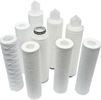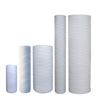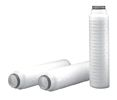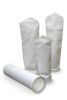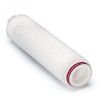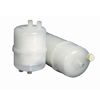1395
com_content.article
(0 votes, average 0 out of 5)
Cách ??c ???ng ??c tuy?n c?a b?m0 out of 50 based on 0 voters.

Cole-Parmer makes every attempt to publish a representative flow versus back pressure curve. In many cases, we can also furnish performance curves that quantify parameters such as NPSHreq.

NPSHreq: The Net Positive Suction Head that must be available to the pump for cavitation-free operation. NPSHreq is typically expressed in either foot of head or units of pressure.Best Efficiency Point

The Best Efficiency Point is the point at which effects of head (pressure) and flow converge to produce the greatest amount of output for the least amount of energy.

NPSHavail = ha - hvpa - hst - hfs when suction lifts fluid

NPSHavail = ha - hvpa + hst - hfs for flooded suction

ha = absolute pressure (in feet of the liquid being pumped) on the surface of the liquid supply level (this will be barometric pressure if suction is from an open tank or sump; or the absolute pressure existing in a closed tank such as a condenser hotwell or deareator).

hvpa = The head in feet corresponding to the vapor pressure of the liquid at the temperature being pumped.

hst = Static height in feet that the liquid supply level is above or below the pump centerline or impeller eye.

hfs = All suction line losses (in feet) including entrance losses and friction losses through pipe, valves, and fittings.

Friction Losses

Friction losses in pipes is commonly calculated with the Darcy-Weisbach equation, in which:

 hf = f x L x V2 D 2g
hf = friction loss in feet of liquid f = friction factor—a dimensionless number which has been determined experimentally and for turbulent flow depends on the roughness of the pipe's interior surface and the Reynolds number. L = pipe length in feet D = average inside diameter of pipe in feet V = average pipe velocity in ft/sec g = gravitational constant (32.174 ft/sec2)
Reynolds Number

The Reynolds number is determined by an equation in which:

 R = VD n
D = inside diameter of pipe in feet
V = average pipe velocity in ft/sec
n = kinematic viscosity in ft2/sec
Friction Factor

In the case of a viscous (laminar) flow, in which the Reynolds number is below 2000, the friction factor is determined by the following equation in which:

 f = 64 R
R = Reynolds number

In the case of turbulent flow, in which the Reynolds number is above 4000, the friction factor can be determined by the following equation developed by C. F. Colebrook:

 ? = [-2 log10 ( ? + 2.51 )] -z 3.7D R√f
? = density at temperature and pressure at which liquid is flowing in lb/ft2
? = absolute roughness (see Pipe Absolute Roughness table below)
D = inside diameter of pipe in feet
R = Reynolds number
f = friction factor
z = absolute or dynamic viscosity in centerpoises

Pipe Absolute Roughness

 Type of pipe Absolute Roughness (?) in feet Drawn tubing (glass, brass, plastic) 0.000005 Commercial steel or wrought iron 0.00015 Cast iron (asphalt dipped) 0.0004 Galvanized iron 0.0005 Cast iron (uncoated) 0.00085 Wood stave 0.0006 to 0.0003 Concrete 0.001 to 0.01 Riveted steel 0.003 to 0.03

Reference source: http://www.coleparmer.com/TechLibraryArticle/629Today 108
Těm ki?m s?n ph?mHŕng có s?n trong kho! Giá t?t khi mua s? l??ng l?n!